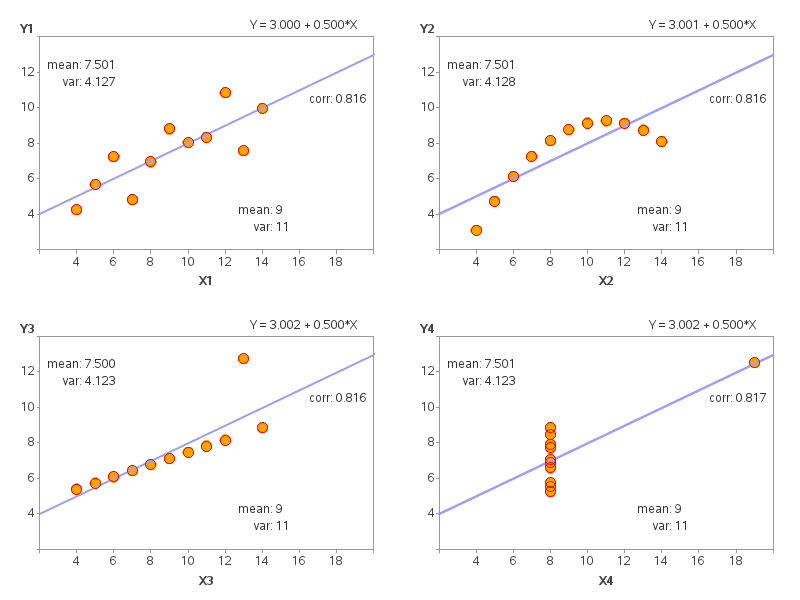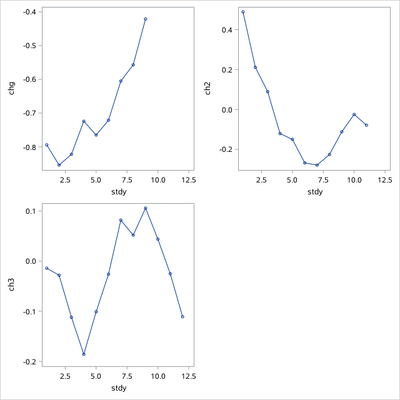## Side-by-Side SG PLOT saved as png

I want all these plots to be saved side-by-side in a PNG file. When I run my code, I get 4 individual PNG files saved for each phase.

Macro:

``````%macro plot1(i=, T=, T7=);
proc sgplot data= &dsn. noautolegend;
where usubjid= "&id." and paramcd = "&param." and aphase = "&i".;
*styleattrs
datacontrastcolors= (red blue orange green)
datalinepatterns= (solid);

%if &T = NA %then %do;
xaxis min= 1 max= 25 values= (1 6 8) valuesdisplay= ("1" "6" "8") label= "Actual CHMI Day";
yaxis min= 0 max= 50 values= (-1 to 2.5 by 0.5) label= "Antibody Reactivity (Baseline-adjusted OD^{unicode '2082'x}410)";
%end;
%else %do;
xaxis min= 1 max= 25 values= (1 6 8 &T. &T7.) valuesdisplay= ("1" "6" "8" "T" "T+7") label= "Actual CHMI Day";
yaxis min= -1 max= 50 values= (-1 to 2.5 by 0.5) label= "Antibody Reactivity (Baseline-adjusted OD410)";
%end;
run;
%mend;
%macro panel(dsn, id, chmi1, chmi17, chmi2, chmi27, chmi3, chmi37, chmi4, chmi47);
ods graphics / reset= index width= 9in height= 7in border= no
imagename= "Fig_immuno_ind_&trt._&id." imagefmt= png;
ods layout gridded columns= 4 advance= table;
title1 "Subject &id."; **try ODS Graphics tool**;

%plot1(i= CHMI1, T= &chmi1., T7= &chmi17.);
%plot1(i= CHMI2, T= &chmi2., T7= &chmi27.);
%plot1(i= CHMI3, T= &chmi3., T7= &chmi37.);
%plot1(i= CHMI4, T= &chmi4., T7= &chmi47.);

ods layout end;
%mend;

%let param= IgG; %let trt= IgG``````

Mock Data:

``````data have;
input usubjid \$ phase \$ stdy paramcd \$ chg trtdy1 trtdy2 @@;
cards;
A	CHMI1	1	IgG 	-0.794	.	.
A	CHMI1	2	IgG 	-0.854	.	.
A	CHMI1	3	IgG 	-0.822	.	.
A	CHMI1	4	IgG 	-0.724	.	.
A	CHMI1	5	IgG 	-0.765	.	.
A	CHMI1	6	IgG 	-0.721	.	.
A	CHMI1	7	IgG 	-0.605	.	.
A	CHMI1	8	IgG 	-0.557	.	.
A	CHMI1	9	IgG 	-0.421	.	.
A	CHMI2	1	IgG 	0.489	.	.
A	CHMI2	2	IgG 	0.211	.	.
A	CHMI2	3	IgG 	0.089	.	.
A	CHMI2	4	IgG 	-0.121	4	11
A	CHMI2	5	IgG 	-0.150	4	11
A	CHMI2	6	IgG 	-0.268	4	11
A	CHMI2	7	IgG 	-0.279	4	11
A	CHMI2	8	IgG 	-0.226	4	11
A	CHMI2	9	IgG 	-0.112	4	11
A	CHMI2	10	IgG 	-0.025	4	11
A	CHMI2	11	IgG 	-0.079	4	11
A	CHMI3	1	IgG 	-0.014	9	.
A	CHMI3	2	IgG 	-0.028	9	.
A	CHMI3	3	IgG 	-0.112	9	.
A	CHMI3	4	IgG 	-0.186	9	.
A	CHMI3	5	IgG 	-0.101	9	.
A	CHMI3	6	IgG 	-0.026	9	.
A	CHMI3	7	IgG 	0.082	9	.
A	CHMI3	8	IgG 	0.052	9	.
A	CHMI3	9	IgG 	0.106	9	.
A	CHMI3	10	IgG 	0.044	9	.
A	CHMI3	11	IgG 	-0.025	9	.
A	CHMI3	12	IgG 	-0.111	9	.
;
run;``````

1 ACCEPTED SOLUTION

Accepted SolutionsDanH_sas
SAS Super FREQ

## Re: Side-by-Side SG PLOT saved as png

Instead of using ODS HTML, use ODS PRINTER with a printer of PNG. Here is a simple example:

``````%macro plot;
ods region;
proc sgplot data=sashelp.class;
vbar age;
run;
%mend;
ods graphics / width=350px;
ods _all_ close;
ods printer printer=png;
ods layout gridded;
ods layout start columns=2;
%plot;
%plot;
%plot;
%plot;
ods layout end;
ods printer close;``````
6 REPLIES 6

## Re: Side-by-Side SG PLOT saved as png

How about providing definitions for the macro variables that you are using but not explaining.

Also your HAVE data does not contain one of the variable used in the plot: PHADY.

Proc SGpanel is intended to place multiple graphs into a single panel, side-by-side or above each other. So might want to consider the procedure used. Panelby variable(s) controls what makes groups of plots.

Since you really aren't providing a lot of options in your SCATTER perhaps you only need Series with the MARKERS option.

## Re: Side-by-Side SG PLOT saved as png

``````* Plot for Individual CHMI Treatment;
%macro plot1(i=, 	/*CHMI treatment number*/
T=, 	/*treatment day*/
T7=	/*7 days post treatment*/
);
proc sgplot data= &dsn. noautolegend;
where usubjid= "&id." and paramcd = "&param." and aphasen = "&i.";
series x= stdy y= chg;
scatter x= stdt y= chg;
*styleattrs
datacontrastcolors= (red blue orange green)
datalinepatterns= (solid);

%if &T = NA %then %do;
xaxis min= 1 max= 25 values= (1 6 8) valuesdisplay= ("1" "6" "8") label= "Actual CHMI Day";
yaxis min= 0 max= 50 values= (-1 to 2.5 by 0.5) label= "Antibody Reactivity (Baseline-adjusted OD^{unicode '2082'x}410)";
%end;
%else %do;
xaxis min= 1 max= 25 values= (1 6 8 &T. &T7.) valuesdisplay= ("1" "6" "8" "T" "T+7") label= "Actual CHMI Day";
yaxis min= -1 max= 50 values= (-1 to 2.5 by 0.5) label= "Antibody Reactivity (Baseline-adjusted OD410)";
%end;
run;
%mend;

* Plots Side-by-Side for Individual Subjects;
%macro panel(dsn, 				/*input dataset*/
id, 				/*subject ID*/
chmi1, chmi17, 	/*CHMI 1 treatment day and 7 days post treatment*/
chmi2, chmi27, 	/*CHMI 2 treatment day and 7 days post treatment*/
chmi3, chmi37, 	/*CHMI 3 treatment day and 7 days post treatment*/
chmi4, chmi47		/*CHMI 4 treatment day and 7 days post treatment*/
);
ods graphics / reset= index width= 9in height= 7in border= no
imagename= "Fig_immuno_ind_&trt._&id." imagefmt= png;
ods layout gridded columns= 4 advance= table;
title1 "Subject &id.";

%plot1(i= CHMI1, T= &chmi1., T7= &chmi17.);
%plot1(i= CHMI2, T= &chmi2., T7= &chmi27.);
%plot1(i= CHMI3, T= &chmi3., T7= &chmi37.);
%plot1(i= CHMI4, T= &chmi4., T7= &chmi47.);

ods layout end;
%mend;

%let param= IgG; %let trt= IgG;

%panel(have, A, NA, NA, 4, 11, 9, NA, NA, NA);
/*Note: subject A only has treatment day available for CHMI 2 and CHMI 3 based on HAVE.TRTDY1*/``````

SGPANEL isn't used since treatment day varies by CHMI. Implementation notes specify non-uniform spacing between CHMI days with treatment day labelled as 'T' and 7-days post treatment labelled as 'T+7'.

## Re: Side-by-Side SG PLOT saved as png

*STDT should be STDY

## Re: Side-by-Side SG PLOT saved as png

If the 4 graphs can share the same axes, I recommend Proc SGpanel.

If you want the 4 graphs to be independent, I recommend Proc GReplay, with 4 Proc GPlot's.

Here's an example of Greplay:DanH_sas
SAS Super FREQ

## Re: Side-by-Side SG PLOT saved as png

Instead of using ODS HTML, use ODS PRINTER with a printer of PNG. Here is a simple example:

``````%macro plot;
ods region;
proc sgplot data=sashelp.class;
vbar age;
run;
%mend;
ods graphics / width=350px;
ods _all_ close;
ods printer printer=png;
ods layout gridded;
ods layout start columns=2;
%plot;
%plot;
%plot;
%plot;
ods layout end;
ods printer close;``````Ksharp
Super User

## Re: Side-by-Side SG PLOT saved as png

Or try PROC SGSCATTER .

```data have;
input usubjid \$ phase \$ stdy paramcd \$ chg trtdy1 trtdy2 @@;
cards;
A CHMI1 1 IgG  -0.794 . .
A CHMI1 2 IgG  -0.854 . .
A CHMI1 3 IgG  -0.822 . .
A CHMI1 4 IgG  -0.724 . .
A CHMI1 5 IgG  -0.765 . .
A CHMI1 6 IgG  -0.721 . .
A CHMI1 7 IgG  -0.605 . .
A CHMI1 8 IgG  -0.557 . .
A CHMI1 9 IgG  -0.421 . .
A CHMI2 1 IgG  0.489 . .
A CHMI2 2 IgG  0.211 . .
A CHMI2 3 IgG  0.089 . .
A CHMI2 4 IgG  -0.121 4 11
A CHMI2 5 IgG  -0.150 4 11
A CHMI2 6 IgG  -0.268 4 11
A CHMI2 7 IgG  -0.279 4 11
A CHMI2 8 IgG  -0.226 4 11
A CHMI2 9 IgG  -0.112 4 11
A CHMI2 10 IgG  -0.025 4 11
A CHMI2 11 IgG  -0.079 4 11
A CHMI3 1 IgG  -0.014 9 .
A CHMI3 2 IgG  -0.028 9 .
A CHMI3 3 IgG  -0.112 9 .
A CHMI3 4 IgG  -0.186 9 .
A CHMI3 5 IgG  -0.101 9 .
A CHMI3 6 IgG  -0.026 9 .
A CHMI3 7 IgG  0.082 9 .
A CHMI3 8 IgG  0.052 9 .
A CHMI3 9 IgG  0.106 9 .
A CHMI3 10 IgG  0.044 9 .
A CHMI3 11 IgG  -0.025 9 .
A CHMI3 12 IgG  -0.111 9 .
;
run;

data want;
merge have(keep=phase stdy chg where=(phase='CHMI1'))
have(keep=phase stdy chg where=(phase2='CHMI2') rename=(phase=phase2 chg=ch2))
have(keep=phase stdy chg where=(phase3='CHMI3') rename=(phase=phase3 chg=ch3)) ;
by stdy;
run;

proc sgscatter data=want;
plot chg*stdy ch2*stdy ch3*stdy/join;
run;```Discussion stats
• 6 replies
• 459 views
• 4 likes
• 5 in conversation